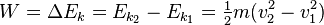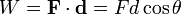# Net Force?

A bullet of mass 20 g strickes a fixed block of wood at a speed of 320m/s. The embeds itself into the block of wood, penetrating to a depth of 6.0cm. Calculate the average net force acting on the bullet while it is being brought to rest.

The block will stop all of the force applied by the bullet; when it just enters the block, the force is equal to that of the force of the bullet, when it stops there is no force. So the average force would be the average of those two forces.

How do I find that force?

Andrew Mason
Homework Helper
The block will stop all of the force applied by the bullet; when it just enters the block, the force is equal to that of the force of the bullet, when it stops there is no force. So the average force would be the average of those two forces.
What is the force of the bullet? By your reasoning, the average force = initial force/2. Why would that be? You seem to be mixing up average velocity with average force.

(Instantaneous) Force = dp/dt = mdv/dt; Average force =?

AM

Well this is a question from a worksheet and it does give the answer, but my teacher wants us to show how we got there! The answer is 1.7 x 10^4. Also How would I find the initial force using those information???

You could apply the Work-Energy Theorem ...

Can you tell me what it is? I don't really remember it!And work can be calculated with the following formula:See if you can put those two together ...

Last edited by a moderator:
What do I substitute the energies with, since I only have a speed of 320m/s? Do sub. that? And the 1/2m(v(2)^2 - v(1)^2 , I don't have v(1)^2!

d is for distance right?

The only unknown is the net force. Read the question again, it says that it is being brought to rest. What does that indicated about the final velocity of the bullet?

And yes, d is distance, in meters.

How do I find the F in W= F*d? Sorry I am over thinking this question! And I am panicking since I still have more physics homework to do!!!!

The work-energy theorem states that work is equal to the change in kinetic energy. However, we also know that work is equal to the net force multiplied by the distance. Therefore:

F/\d = 1/2m(v2^2 - v1^2).

To solve for the net force, just divide by the distance.

F = (1/2m(V2^2 - v1^2))/(/\d)

But since the final velocity is zero:

F = (1/2m(-v1^2))/(/\d).

So make sure all the units are correct, substitute and done.

OMG....YOU ARE THE BEST! Even though i don't know you! THANKS A BUNCH. Can you also check out my other post. I haven't gotten any help for that.....!!!

Wait what is v1???

The initial speed, before it hits the block. 320m/s.

Oh ok.